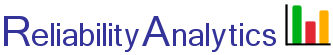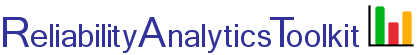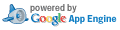# Exponential Distribution

The exponential distribution is often used to model the reliability of electronic systems, which do not typically experience wearout type failures. The distribution is called "memoryless," meaning that the calculated reliability for say, a 10 hour mission, is the same for a subsequent 10 hour mission, given that the system is working properly at the start of each mission. Given a hazard (failure) rate, λ, or mean time between failure (MTBF=1/λ), the reliability can be determined at a specific point in time (t). The exponential distribution probability density function, reliability function and hazard rate are given by: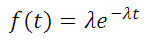Probability Density FunctionReliability Function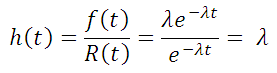Hazard Rate

For example, given an electronic system with a mean time between failure of 700 hours, the reliability at the t=700 hour point is 0.37, as represented by the green shaded area in the picture below. Therefore, if a system fails in accordance with the exponential distribution, there is only a 37% chance of failure-free operation for a length of time equal to its MTBF.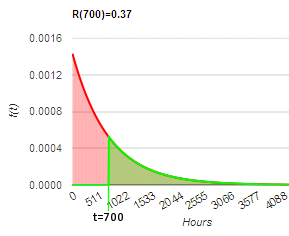## Calculation Inputs:

1.
2. Time period of interest (t):
3. Units associated with inputs #2 and #3 above:
4. Decimal places:
5. Options:

Table
f(t) chart
R(t) chart
F(t) chart
h(t) chart

Title:

## Featured Reference:An Introduction to Reliability and Maintainability Engineering

Toolkit Home